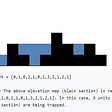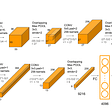# Deep Learning

A baby learns to crawl, walk and then run. We are in the crawling stage when it comes to applying machine learning.” ~Dave Waters

Artificial Intelligent or AI is most important topic in today’s world. By using AI we make our computer/system make intelligent to think ,observe ,understand and then predict about the things.

when we talk about AI then Machine Learning & Deep Learning both are very important part of AI ,without this AI is incomplete.

Machine Learning is developments of computer programs require data and use it learn for themselves are the main features of Machine learning. The procedure of learning begins with observations such as examples, instruction, or direct experience, in order to look for patterns in data and make enhanced decisions on the examples that we provide for the future based enhancement. The most important aim is to allow computers to learn without human assistance or intervention and adjust actions accordingly.

Machine Learning based Algorithms are known as Supervised and Unsupervised Learning.

Supervised machine learning algorithms are used to predict future events based on what has been learned or we need to train the data using algorithms , the concepts of Simple -Linear Regression and Multi-Linear Regression,Binary and Multi classification. Starting from the analysis of a known training dataset,selecting features ,eliminating features,getting accuracy in the model(training dataset ), an inferred function to make predictions about the output values etc. are important steps of this algorithm.

In comparison, unsupervised machine learning algorithms are exercised when the information used to instruct is neither labeled nor classified. Machine learning which is unsupervised studies how systems can deduce a function to describe a concealed arrangement from unlabeled data. The system explores the data and can draw inferences from datasets to describe hidden structures from unlabeled data but not always figures out the right output. Amazon, Google, Salesforce, Netflix and IBM are some of the well-known names who are nailing the Machine Learning thing.

Another incredible subfield of AI is Deep Learning.

“Incorporating general intelligence, bodily intelligence, emotional intelligence, spiritual intelligence, political intelligence and social intelligence in AI systems are part of the future deep learning research. ”

Deep learning or DL is concerned with structure & functionality of brain.We can think like we the humans have neurons inside the brain which are information messengers. They use electrical impulses and chemical signals to transmit information between different areas of the brain, and between the brain and the rest of the nervous system. Just like in DL we hear the term deep learning, we just think of a large deep neural net. In deep learning “Deep” refers to the number of layers typically and so this kind of the popular term that’s been adopted in the press. Everybody thinks of it as deep artificial neural networks. The significant property of neural networks is that their results get better with more data, more computation, and bigger models . In addition to scalability, another often quoted benefit of deep learning models is their capability to execute automatic feature extraction from raw data, also called feature learning. Deep learning algorithms seek to exploit the unknown structure in the input allocation in order to determine good representations, often at higher levels, with multiple-level learned attributes defined in terms of lower-level features.

Let’s discuss the different types of neural networks that are used to solve deep learning problems.

## Artificial Neural Networks (ANN)

As you can see ANN consists of three layers i.e. INPUT,HIDDEN & OUTPUT layers.ANN can be used to solve problems related to:

• Tabular data
• Image data
• Text data

Key Points related to the architecture:

1. The network architecture has an input layer, hidden layer (there can be more than 1) and the output layer. It is also called MLP (Multi Layer Perceptron) because of the multiple layers.

2. The hidden layer can be seen as a “distillation layer” that distills some of the important patterns from the inputs and passes it onto the next layer to see. It makes the network faster and efficient by identifying only the important information from the inputs leaving out the redundant information

3. The activation function serves two notable purposes:

- It captures non-linear relationship between the inputs

- It helps convert the input into a more useful output.

In the above example, the activation function used is sigmoid:

O1 = 1 / (1+exp(-F))

Where F = W1*X1 + W2*X2 + W3*X3

Sigmoid activation function creates an output with values between 0 and 1. There can be other activation functions like Tanh, softmax and RELU.

4. Similarly, the hidden layer leads to the final prediction at the output layer:

O3 = 1 / (1+exp(-F 1))

Where F 1= W7*H1 + W8*H2

Here, the output value (O3) is between 0 and 1. A value closer to 1 (e.g. 0.75) indicates that there is a higher indication of customer defaulting.

5. The weights W are the importance associated with the inputs. If W1 is 0.56 and W2 is 0.92, then there is higher importance attached to X2: Debt Ratio than X1: Age, in predicting H1.

6. The above network architecture is called “feed-forward network”, as you can see that input signals are flowing in only one direction (from inputs to outputs). We can also create “feedback networks where signals flow in both directions.

7. A good model with high accuracy gives predictions that are very close to the actual values.

8. The key to get a good model with accurate predictions is to find “optimal values of W — weights” that minimizes the prediction error. This is achieved by “Back propagation algorithm” and this makes ANN a learning algorithm because by learning from the errors, the model is improved.

9. The most common method of optimization algorithm is called “gradient descent”, where, iteratively different values of W are used and prediction errors assessed. So, to get the optimal W, the values of W are changed in small amounts and the impact on prediction errors assessed. Finally, those values of W are chosen as optimal, where with further changes in W, errors are not reducing further.

Forecasting ,Image Processing and Character recognition are the application of ANN

## Convolution Neural Networks (CNN)

Overview of CNN layers

first convolution layer captures simple features while the last convolution layer captures complex features of training samples.

The building blocks of CNNs are filters a.k.a. kernels. Kernels are used to extract the relevant features from the input using the convolution operation. Let’s try to grasp the importance of filters using images as input data.

• CNN also follows the concept of parameter sharing. A single filter is applied across different parts of an input to produce a feature map:

Convolving image with a filter

Notice that the 2*2 feature map is produced by sliding the same 3*3 filter across different parts of an image.

Key-points:

1. The convolution output is then passed through an activation unit called ReLU (Rectified Linear Unit). This unit converts the data into its non-linear form. The output of ReLU is clipped to zero only if convolution output is negative. Sigmoid units are not preferred as activation unit because of vanishing gradient problem. If the depth of CNN is large, then by the time the gradient found at the input layer traverses to the output layer, it’s value would have diminished largely. This results in the overall output of the network varying marginally. This, in turn, results in slow/no convergence. To avoid such a situation, ReLU is preferred.
2. The output of ReLU is then passed through a pooling layer. Pooling layer remove any redundant features that’s captured during convolution. Thus, this layer reduces the size of data sample. The principle behind pooling is that it assumes that adjacent values of image pixels are nearly identical. The average/minimum/maximum of four adjacent pixel values are used to carry out pooling. In general, size of input image is reduced by half with help of a 2*2 filter. The input data may/ may not be subjected to zero padding before pooling.
3. Dropout layer is used to reduce over-fitting by making the CNN model robust to noise. These layers are generally introduced between 2 fully connected neural network layers. They temporarily cut a portion of data flowing between two fully connected layers. This is equivalent to making the model learn to classify accurately in presence of noise. Thus, chances of model classifying inaccurately because of overfitting is reduced.
4. The output of CNN model is calculated using SoftMax function. SoftMax is preferred as it gives the probability of outputs for different classes rather than just >= 0.5 in the case of sigmoid output. The usage of SoftMax function to find output results based on the highest probability of class results in an increase in accuracy the of output.
5. Cross entropy is used to measure the performance of the system. They are calculated with help of a SoftMax function. The advantage here is that the SoftMax output is the trace of the elements corresponding to the class that we know that the output belongs too. This, in general, saves the computation time.

## Recurrent Neural Networks (RNN)

We can use recurrent neural networks to solve the problems related to:

• Time Series data
• Text data
• Audio data
• RNN captures the sequential information present in the input data i.e. dependency between the words in the text while making predictions:

As you can see here, the output (o1, o2, o3, o4) at each time step depends not only on the current word but also on the previous words.

# Comparing the Different Types of Neural Networks (MLP(ANN) vs. RNN vs. CNN)

Here, I have summarized some of the differences among different types of neural networks:

## More from Rachana Jaiswal

Love podcasts or audiobooks? Learn on the go with our new app.

## COVID19, Mental Health & Artificial Intelligence — Part2## The Keigo FAQ## Spice It Up: How AI can be Used for Small Business Opportunities## It’s Time to Call B.S. on the Metaverse. It Does Not Exist. Yet.## What is Edge AI and why is it important## The rise of AI operators, dynamic parking meters and Amazon Echo as a crime fighter— This Week’s 10…## Trapping Rain Water## AlexNet Architecture